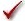## Please enable JavaScript to view this site.

 Navigation: PRINCIPLES OF STATISTICS > Analysis checklists Analysis checklist: Unpaired t test

The unpaired t test compares the means of two unmatched groups, assuming that the values follow a Gaussian distribution. Read elsewhere to learn about choosing a t test, and interpreting the results.

###Are the populations distributed according to a Gaussian distribution?

The unpaired t test assumes that you have sampled your data from populations that follow a Gaussian distribution. This assumption matters less with large samples due to the Central Limit Theorem.

Prism can perform normality tests as part of the Column Statistics analysis. Learn more.

If your data do not come from Gaussian distributions, you have three options. Your best option is to transform the values (perhaps to logs or reciprocals) to make the distributions more Gaussian. Another choice is to use the Mann-Whitney nonparametric test instead of a t test. A final option is to use t test anyway, knowing that it is fairly robust to violations of a Gaussian distribution with large samples.

###Do the two populations have the same variances?

The unpaired t test assumes that the two populations have the same variances (and thus the same standard deviation).

Prism tests for equality of variance with an F test. The P value from this test answers this question: If the two populations really have the same variance, what is the chance that you would randomly select samples whose ratio of variances is as far from 1.0 (or further) as observed in your experiment? A small P value suggests that the variances are different.

Don't base your conclusion solely on the F test. Also think about data from other similar experiments. If you have plenty of previous data that convinces you that the variances are really equal, ignore the F test (unless the P value is really tiny) and interpret the t test results as usual.

In some contexts, finding that populations have different variances may be as important as finding different means.

###Are the data unpaired?

The unpaired t test works by comparing the difference between means with the standard error of the difference, computed by combining the standard errors of the two groups. If the data are paired or matched, then you should choose a paired t test instead. If the pairing is effective in controlling for experimental variability, the paired t test will be more powerful than the unpaired test.

###Are the “errors” independent?

The term “error” refers to the difference between each value and the group mean. The results of a t test only make sense when the scatter is random – that whatever factor caused a value to be too high or too low affects only that one value. Prism cannot test this assumption. You must think about the experimental design. For example, the errors are not independent if you have six values in each group, but these were obtained from two animals in each group (in triplicate). In this case, some factor may cause all triplicates from one animal to be high or low.

###Are you comparing exactly two groups?

Use the t test only to compare two groups. To compare three or more groups, use one-way ANOVA followed by multiple comparison tests. It is not appropriate to perform several t tests, comparing two groups at a time. Making multiple comparisons increases the chance of finding a statistically significant difference by chance and makes it difficult to interpret P values and statements of statistical significance. Even if you want to use planned comparisons to avoid correcting for multiple comparisons, you should still do it as part of one-way ANOVA to take advantage of the extra degrees of freedom that brings you.

###Do both columns contain data?

If you want to compare a single set of experimental data with a theoretical value (perhaps 100%) don't fill a column with that theoretical value and perform an unpaired t test. Instead, use a one-sample t test.

###Do you really want to compare means?

The unpaired t test compares the means of two groups. It is possible to have a tiny P value – clear evidence that the population means are different – even if the two distributions overlap considerably. In some situations – for example, assessing the usefulness of a diagnostic test – you may be more interested in the overlap of the distributions than in differences between means.

###If you chose a one-tail P value, did you predict correctly?

If you chose a one-tail P value, you should have predicted which group would have the larger mean before collecting any data. Prism does not ask you to record this prediction, but assumes that it is correct. If your prediction was wrong, then ignore the P value reported by Prism and state that P>0.50.